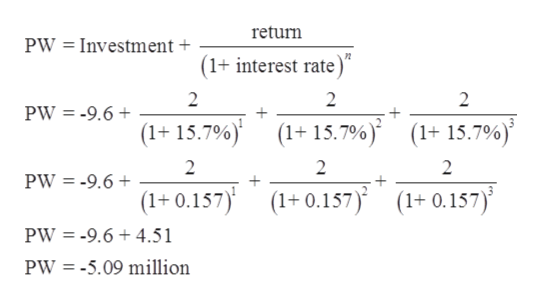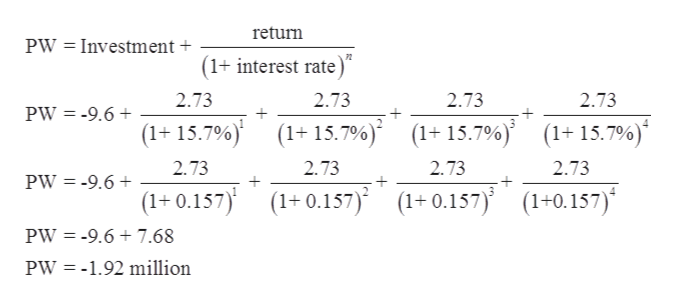# "A corporation is trying to decide whether to buy the patent for a product designed by another company. The decision to buy will mean an investment of \$9.6 million, and the demand for the product is not known. If demand is light, the company expects a return of \$2 million each year for the first three years and no return in the fourth year. If demand is moderate, the return will be \$2.73 million each year for four years, and high demand means a return of \$5.4 million each year for four years. It is estimated the probability of a high demand is 0.47, and the probability of a light demand is 0.21. The firm's interest rate is 15.7%.Calculate the expected present worth of the patent. Express your answer in millions of dollars. For example, if the answer is \$12.3 million, enter 12.3. (All figures represent after-tax values.)"

Question
6 views

"A corporation is trying to decide whether to buy the patent for a product designed by another company. The decision to buy will mean an investment of \$9.6 million, and the demand for the product is not known. If demand is light, the company expects a return of \$2 million each year for the first three years and no return in the fourth year. If demand is moderate, the return will be \$2.73 million each year for four years, and high demand means a return of \$5.4 million each year for four years. It is estimated the probability of a high demand is 0.47, and the probability of a light demand is 0.21. The firm's interest rate is 15.7%.
Calculate the expected present worth of the patent. Express your answer in millions of dollars. For example, if the answer is \$12.3 million, enter 12.3. (All figures represent after-tax values.)"

check_circle

Step 1

Given: Interest rate = 15.7%

Probability of high demand = 0.47

Probability of light demand = 0.21

Step 2

Present worth when there is light demand.help_outlineImage Transcriptionclosereturn PW = Investment + (1+ interest rate)" 2 PW = -9.6 + (1+ 15.7%) (1+ 15.7%) (1+ 15.7%)' 2 2 PW = -9.6 + (1+ 0.157) (1+ 0.157) (1+ 0.157) PW = -9.6 + 4.51 PW = -5.09 million %3D fullscreen
Step 3

Present worth when there i...help_outlineImage Transcriptionclosereturn PW = Investment + (1+ interest rate)" 2.73 2.73 2.73 2.73 PW = -9.6 + (1+ 15.7%) (1+ 15.7%) (1+15.7%)* (1+ 15.7%)' 2.73 2.73 2.73 2.73 PW = -9.6 + (1+ 0.157)' (1+ 0.157) (1+ 0.157) (1+0.157)* PW = -9.6 + 7.68 PW = -1.92 million fullscreen

### Want to see the full answer?

See Solution

#### Want to see this answer and more?

Solutions are written by subject experts who are available 24/7. Questions are typically answered within 1 hour.*

See Solution
*Response times may vary by subject and question.
Tagged in